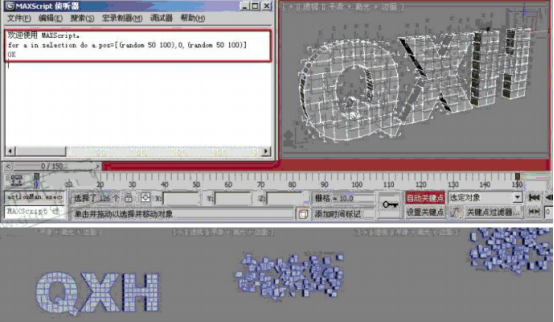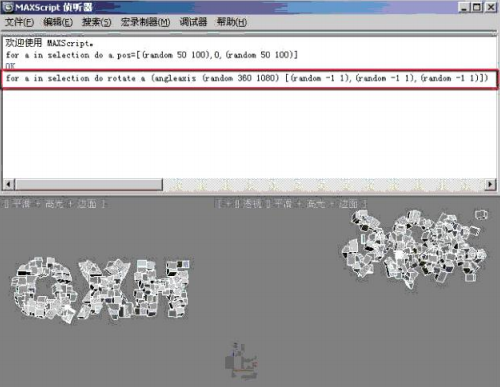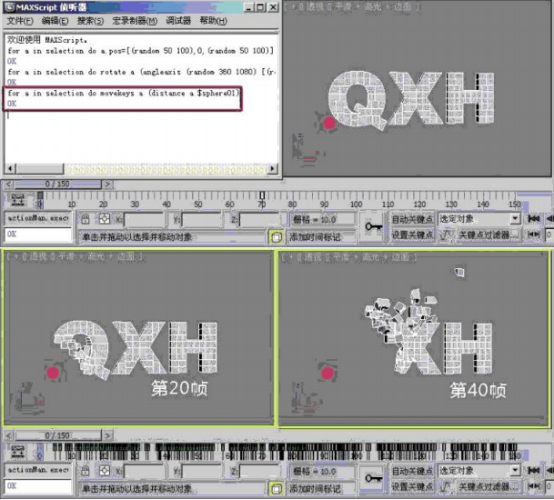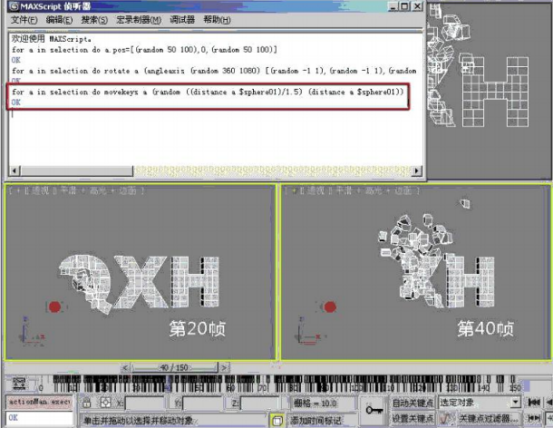×# 3dmax文字破碎效果动画教程（三）飞散动画

【第三部分:利用脚本制作飞散动画】

➊制作位移动画

for a in selection do a.pos=[(random 50 100),0,(random 50 100)]❷制作旋转动画

for a in selection do rotate a (angleaxis (random 360 1080)[(random-1 1),(random -1 1),(random-1 1)])❸偏移动画

for a in selection do movekeys a (distance a \$sphere01)❹优化偏移效果

for a in selection do movekeys a (random ((distance a \$sphere01)/1.5)(distance a \$sphere01))